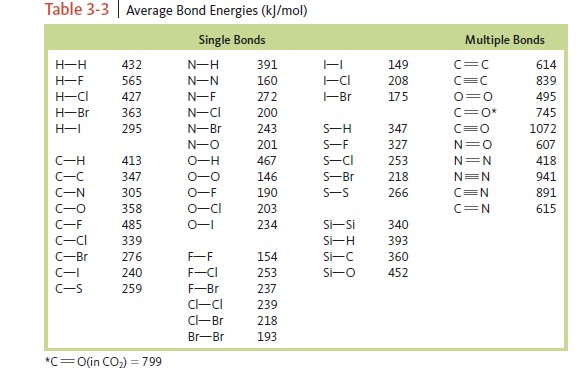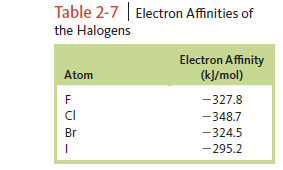# Problem: Use bond energies (Table 3‑3), values of electron affinities (Table 2-7), and the ionization energy of hydrogen (1312 kJ/mol) to estimate ΔE for each of the following reactions.c. HI (g) → H+ (g) + I - (g)

###### FREE Expert Solution

HI (g) → H+ (g) + I - (g)

84% (426 ratings)###### Problem Details

Use bond energies (Table 3‑3), values of electron affinities (Table 2-7), and the ionization energy of hydrogen (1312 kJ/mol) to estimate ΔE for each of the following reactions.

c. HI (g) → H+ (g) + I - (g)